## 1. 类和对象

### 1.1 类和对象的理解

• 类的理解
• 类是对现实生活中一类具有共同属性和行为的事物的抽象
• 类是对象的数据类型，类是具有相同属性和行为的一组对象的集合
• 简单理解：类就是对现实事物的一种描述
• 类的组成
• 属性：指事物的特征，例如：手机事物（品牌，价格，尺寸）
• 行为：指事物能执行的操作，例如：手机事物（打电话，发短信）
• 类和对象的关系
• 类：类是对现实生活中一类具有共同属性和行为的事物的抽象
• 对象：是能够看得到摸的着的真实存在的实体
• 简单理解：类是对事物的一种描述，对象则为具体存在的事物

### 1.2 类的定义

• 属性：在类中通过成员变量来体现（类中方法外的变量）
• 行为：在类中通过成员方法来体现（和前面的方法相比去掉static关键字即可）

①定义类

②编写类的成员变量

③编写类的成员方法

``````public class 类名 {

// 成员变量
变量1的数据类型 变量1；
变量2的数据类型 变量2;
…
// 成员方法
方法1;
方法2;
}
``````

``````/*
手机类：
类名：
手机(Phone)

成员变量：
品牌(brand)
价格(price)

成员方法：
打电话(call)
发短信(sendMessage)
*/
public class Phone {

//成员变量
String brand;
int price;

//成员方法
public void call() {

System.out.println("打电话");
}

public void sendMessage() {

System.out.println("发短信");
}
}

``````

### 1.3 对象的使用

• 创建对象的格式：
• 类名 对象名 = new 类名();
• 调用成员的格式：
• 对象名.成员变量
• 对象名.成员方法();
• 示例代码
``````/*
创建对象
格式：类名 对象名 = new 类名();
范例：Phone p = new Phone();

使用对象
1：使用成员变量
格式：对象名.变量名
范例：p.brand
2：使用成员方法
格式：对象名.方法名()
范例：p.call()
*/
public class PhoneDemo {

public static void main(String[] args) {

//创建对象
Phone p = new Phone();

//使用成员变量
System.out.println(p.brand);
System.out.println(p.price);

p.brand = "小米";
p.price = 2999;

System.out.println(p.brand);
System.out.println(p.price);

//使用成员方法
p.call();
p.sendMessage();
}
}
``````

### 1.4 学生对象-练习

• 需求：首先定义一个学生类，然后定义一个学生测试类，在学生测试类中通过对象完成成员变量和成员方法的使用
• 分析：
• 成员变量：姓名，年龄…
• 成员方法：学习，做作业…
• 示例代码：
``````!(C:\TeachRes\Java2019\JavaSe01\day07\4-笔记\笔记图片资源包\1.png)class Student {

//成员变量
String name;
int age;

//成员方法
public void study() {

System.out.println("好好学习，天天向上");
}

public void doHomework() {

System.out.println("键盘敲烂，月薪过万");
}
}
/*
学生测试类
*/
public class StudentDemo {

public static void main(String[] args) {

//创建对象
Student s = new Student();

//使用对象
System.out.println(s.name + "," + s.age);

s.name = "林青霞";
s.age = 30;

System.out.println(s.name + "," + s.age);

s.study();
s.doHomework();
}
}
``````

## 2. 对象内存图

### 2.1 单个对象内存图

• 成员变量使用过程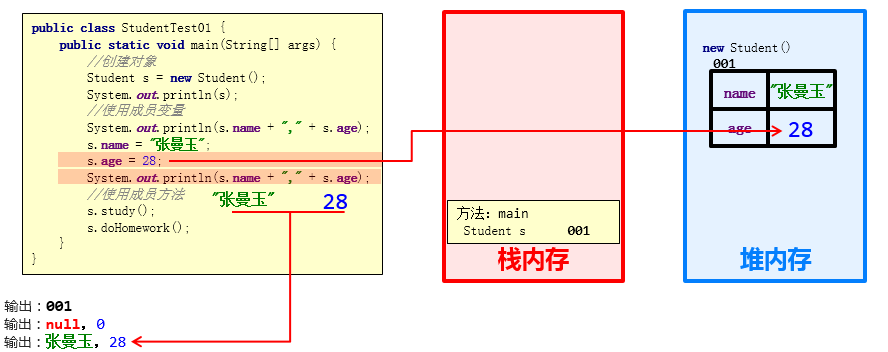• 成员方法调用过程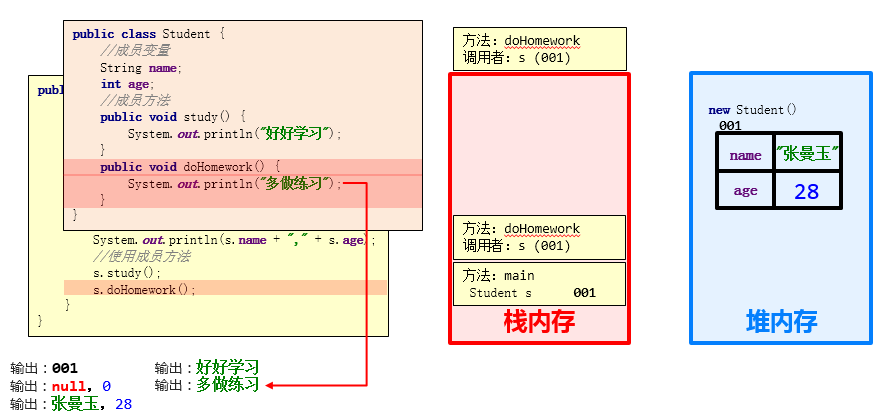### 2.2 多个对象内存图

• 成员变量使用过程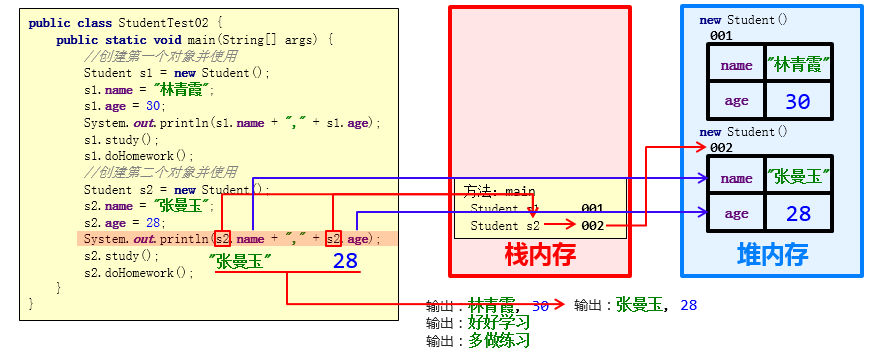• 成员方法调用过程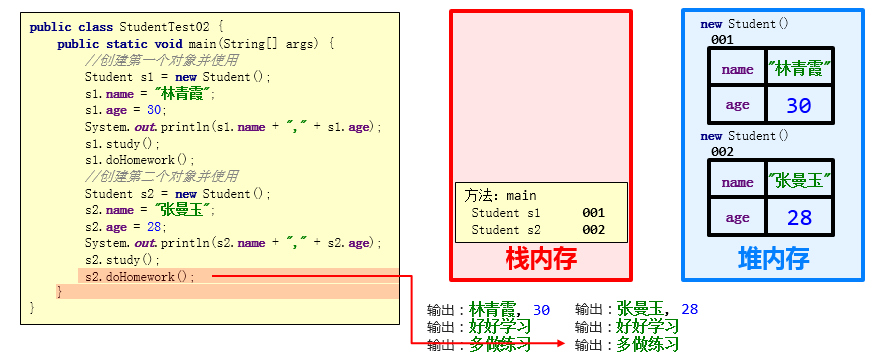• 总结：

多个对象在堆内存中，都有不同的内存划分，成员变量存储在各自的内存区域中，成员方法多个对象共用的一份

### 2.3 多个对象指向相同内存图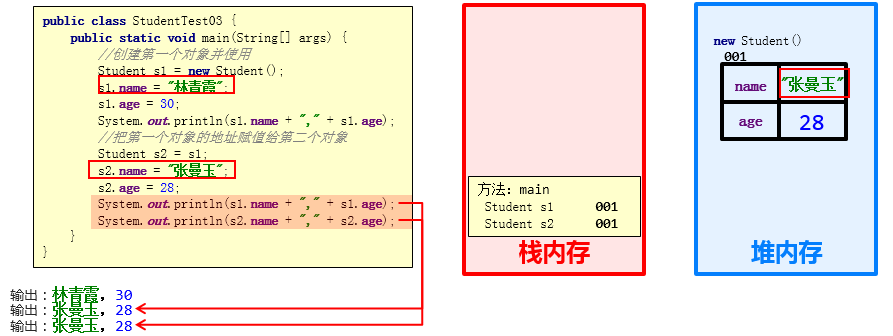• 总结

当多个对象的引用指向同一个内存空间（变量所记录的地址值是一样的）

只要有任何一个对象修改了内存中的数据，随后，无论使用哪一个对象进行数据获取，都是修改后的数据。

## 3. 成员变量和局部变量

### 3.1 成员变量和局部变量的区别

• 类中位置不同：成员变量（类中方法外）局部变量（方法内部或方法声明上）
• 内存中位置不同：成员变量（堆内存）局部变量（栈内存）
• 生命周期不同：成员变量（随着对象的存在而存在，随着对象的消失而消失）局部变量（随着方法的调用而存在，醉着方法的调用完毕而消失）
• 初始化值不同：成员变量（有默认初始化值）局部变量（没有默认初始化值，必须先定义，赋值才能使用）

## 4. 封装

### 4.1 private关键字

private是一个修饰符，可以用来修饰成员（成员变量，成员方法）

• 被private修饰的成员，只能在本类进行访问，针对private修饰的成员变量，如果需要被其他类使用，提供相应的操作
• 提供“get变量名()”方法，用于获取成员变量的值，方法用public修饰
• 提供“set变量名(参数)”方法，用于设置成员变量的值，方法用public修饰
• 示例代码：

``````/*
学生类
*/
class Student {

//成员变量
String name;
private int age;

//提供get/set方法
public void setAge(int a) {

if(a<0 || a>120) {

System.out.println("你给的年龄有误");
} else {

age = a;
}
}

public int getAge() {

return age;
}

//成员方法
public void show() {

System.out.println(name + "," + age);
}
}
/*
学生测试类
*/
public class StudentDemo {

public static void main(String[] args) {

//创建对象
Student s = new Student();
//给成员变量赋值
s.name = "林青霞";
s.setAge(30);
//调用show方法
s.show();
}
}
``````

### 4.2 private的使用

• 需求：定义标准的学生类，要求name和age使用private修饰，并提供set和get方法以及便于显示数据的show方法，测试类中创建对象并使用，最终控制台输出 林青霞，30

• 示例代码：

``````/*
学生类
*/
class Student {

//成员变量
private String name;
private int age;

//get/set方法
public void setName(String n) {

name = n;
}

public String getName() {

return name;
}

public void setAge(int a) {

age = a;
}

public int getAge() {

return age;
}

public void show() {

System.out.println(name + "," + age);
}
}
/*
学生测试类
*/
public class StudentDemo {

public static void main(String[] args) {

//创建对象
Student s = new Student();

//使用set方法给成员变量赋值
s.setName("林青霞");
s.setAge(30);

s.show();

//使用get方法获取成员变量的值
System.out.println(s.getName() + "---" + s.getAge());
System.out.println(s.getName() + "," + s.getAge());

}
}
``````

### 4.3 this关键字

• this修饰的变量用于指代成员变量，其主要作用是（区分局部变量和成员变量的重名问题）

• 方法的形参如果与成员变量同名，不带this修饰的变量指的是形参，而不是成员变量
• 方法的形参没有与成员变量同名，不带this修饰的变量指的是成员变量
``````public class Student {

private String name;
private int age;

public void setName(String name) {

this.name = name;
}

public String getName() {

return name;
}

public void setAge(int age) {

this.age = age;
}

public int getAge() {

return age;
}

public void show() {

System.out.println(name + "," + age);
}
}
``````

### 4.4 this内存原理

• this代表当前调用方法的引用，哪个对象调用的方法，this就代表哪一个对象

• 示例代码：

``````public class StudentDemo {

public static void main(String[] args) {

Student s1 = new Student();
s1.setName("林青霞");
Student s2 = new Student();
s2.setName("张曼玉");
}
}
``````
• 图解：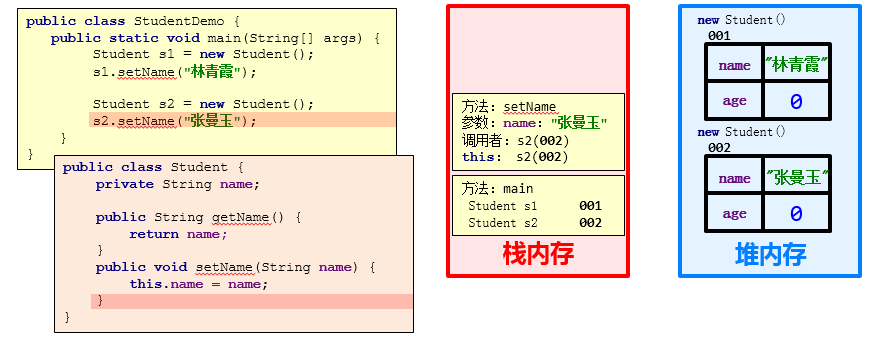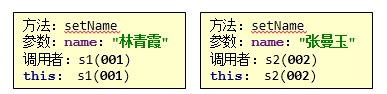### 4.5 封装思想

1. 封装概述
是面向对象三大特征之一（封装，继承，多态）
是面向对象编程语言对客观世界的模拟，客观世界里成员变量都是隐藏在对象内部的，外界是无法直接操作的
2. 封装原则
将类的某些信息隐藏在类内部，不允许外部程序直接访问，而是通过该类提供的方法来实现对隐藏信息的操作和访问
成员变量private，提供对应的getXxx()/setXxx()方法
3. 封装好处
通过方法来控制成员变量的操作，提高了代码的安全性
把代码用方法进行封装，提高了代码的复用性

## 5. 构造方法

### 5.1 构造方法概述

• 作用：创建对象 Student stu = new Student();

• 格式：

public class 类名{

​ 修饰符 类名( 参数 ) {

​ }

}

• 功能：主要是完成对象数据的初始化

• 示例代码：
``````class Student {

private String name;
private int age;

//构造方法
public Student() {

System.out.println("无参构造方法");
}

public void show() {

System.out.println(name + "," + age);
}
}
/*
测试类
*/
public class StudentDemo {

public static void main(String[] args) {

//创建对象
Student s = new Student();
s.show();
}
}
``````

### 5.2 构造方法的注意事项

• 构造方法的创建

• 构造方法的重载

• 推荐的使用方式

• 重要功能！

• 示例代码
``````/*
学生类
*/
class Student {

private String name;
private int age;

public Student() {

}

public Student(String name) {

this.name = name;
}

public Student(int age) {

this.age = age;
}

public Student(String name,int age) {

this.name = name;
this.age = age;
}

public void show() {

System.out.println(name + "," + age);
}
}
/*
测试类
*/
public class StudentDemo {

public static void main(String[] args) {

//创建对象
Student s1 = new Student();
s1.show();

//public Student(String name)
Student s2 = new Student("林青霞");
s2.show();

//public Student(int age)
Student s3 = new Student(30);
s3.show();

//public Student(String name,int age)
Student s4 = new Student("林青霞",30);
s4.show();
}
}
``````

### 5.3 标准类制作

①类名需要见名知意

②成员变量使用private修饰

③提供至少两个构造方法

• 无参构造方法

• 带全部参数的构造方法

④成员方法

• 提供每一个成员变量对应的setXxx()/getXxx()

• 如果还有其他行为，也需要写上

## 6.练习1-定义学生类

​ 定义标准学生类，要求分别使用空参和有参构造方法创建对象，空参创建的对象通过setXxx赋值，有参创建的对象直接赋值，并通过show方法展示数据。

``````class Student {

//成员变量
private String name;
private int age;

//构造方法
public Student() {

}

public Student(String name, int age) {

this.name = name;
this.age = age;
}

//成员方法
public void setName(String name) {

this.name = name;
}

public String getName() {

return name;
}

public void setAge(int age) {

this.age = age;
}

public int getAge() {

return age;
}

public void show() {

System.out.println(name + "," + age);
}
}
/*
创建对象并为其成员变量赋值的两种方式
1:无参构造方法创建对象后使用setXxx()赋值
2:使用带参构造方法直接创建带有属性值的对象
*/
public class StudentDemo {

public static void main(String[] args) {

//无参构造方法创建对象后使用setXxx()赋值
Student s1 = new Student();
s1.setName("林青霞");
s1.setAge(30);
s1.show();

//使用带参构造方法直接创建带有属性值的对象
Student s2 = new Student("林青霞",30);
s2.show();
}
}
``````

## 7.练习2-创建对象并添加到数组

​ 定义一个标准的javabean类
​ Student学生类
​ 属性：name，age
​ 在测试类中，创建两个学生对象并添加到数组中。

``````public class Test {

public static void main(String[] args) {

//需求：
//定义一个标准的javabean类
//Student学生类
//属性：name，age
//在测试类中，创建两个学生对象
Student s1 = new Student();
s1.setName("zhangsan");
s1.setAge(23);

Student s2 = new Student("lisi", 24);

//创建一个长度为2的数组，用来存储学生对象
Student[] arr = new Student;

//把学生对象添加到数组当中
arr = s1;
arr = s2;

//遍历数组，打印两个学生对象的所有信息
for (int i = 0; i < arr.length; i++) {

//i 索引   arr[i] 元素（学生对象）
Student stu = arr[i];
System.out.println(stu.getName() + ", " + stu.getAge());
}

}
}

public class Student {

private String name;
private int age;

//空参构造
//带全部参数的构造
//针对于每一个私有化的成员变量都要提供对有的get和set方法
public Student() {

}

public Student(String name, int age) {

this.name = name;
this.age = age;
}

public String getName() {

return name;
}

public void setName(String name) {

this.name = name;
}

public int getAge() {

return age;
}

public void setAge(int age) {

this.age = age;
}
}

``````

## 8.练习3-修改年龄

​ 定义javabean类，要求如下：

​ 学生类（属性：姓名、年龄）

​ 在测试类中，创建5个学生对象并添加到数组中

​ 再遍历数组，如果学生的年龄小于18岁，那么就在原有基础上+2岁

​ 最后遍历数组

``````public class Test {

public static void main(String[] args) {

//1.需求：
//定义javabean类(Student)
//属性：姓名，年龄
//2.定义五个学生对象，
Student s1 = new Student("zhangsan",23);
Student s2 = new Student("lisi",15);
Student s3 = new Student("wangwu",17);
Student s4 = new Student("zhaoliu",21);
Student s5 = new Student("qianqi",22);
//3.添加到数组当中
Student[] arr = new Student;
arr = s1;
arr = s2;
arr = s3;
arr = s4;
arr = s5;
//4.如果学生的年龄小于18岁，那么就在原有的基础上+2岁。
for (int i = 0; i < arr.length; i++) {

//i 索引 arr[i] 元素(学生对象)
Student stu = arr[i];
int age = stu.getAge();
if(age < 18){

//如果小于18，我们就要在原有的基础上+2
//age + 2;
stu.setAge(age + 2);
}
}
//5.遍历数组
for (int i = 0; i < arr.length; i++) {

Student stu = arr[i];
System.out.println(stu.getName() +", " + stu.getAge());
}
}
}

package com.itheima.demo9;

public class Student {

private String name;
private int age;

public Student() {

}

public Student(String name, int age) {

this.name = name;
this.age = age;
}

/**
* 获取
* @return name
*/
public String getName() {

return name;
}

/**
* 设置
* @param name
*/
public void setName(String name) {

this.name = name;
}

/**
* 获取
* @return age
*/
public int getAge() {

return age;
}

/**
* 设置
* @param age
*/
public void setAge(int age) {

this.age = age;
}

}
``````

## 9.快速生成javabean的方式

1，先定义javabean中的属性

2，在javabean类的空白处，按快捷键：

​ alt + Insert 或者 alt + Fn + Insert

3，选择第一个，生成构造方法

4，选择get and set 生成get和set方法

## 10.八大Java常用类

Java的常用类哟如下几种: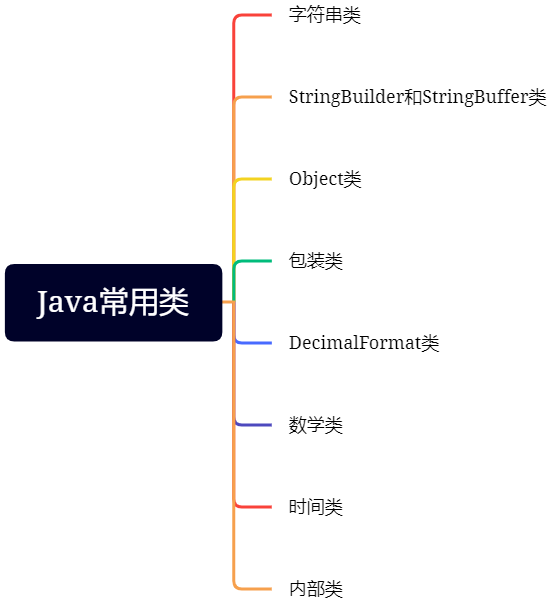### 10.1 字符串类

char charAt() 获取某个位置的字符
String concat() 字符串的拼接。一般字符串拼接直接相加就好了
boolean contains() 判断原字符串是否含有某个字符串，常用于子串的判断
boolean endsWith() 判断原字符串是否以某个字符串结尾
boolean startsWith() 判断原字符串是否以某个字符串开头
boolean equals() 判断两边字符串内容是否相同；==判断地址是否相同
boolean equalsIgnoreCase() 忽略大小写判断两边字符串的内容是否一样
int indexOf() 计算给出字符串第一个出现的位置
int LastindexOf() 计算给出字符串最后一个出现的位置
int length() 计算字符串的长度
String replace() 字符串内容的替换
String[] split() 字符串切割，最终结果是一个字符串数组
String substring() 字符串截取，左闭右开：[ )
String trim() 去掉字符串左右两边的空格，中间的不行
static String valueOf() 官方：基本数据类型转为字符串操作；直接：变量 + “”

``````public class Test {

public static void main(String[] args) {

String s = "我的名字叫每天都要努力的小颓废呀";

s.concat("hhh"); // 在字符串s上拼接，拼接hhh
System.out.println(s);// 我的名字叫李华
//字符串是不可变的数据类型
//几乎所有的字符串操作都会返回一个新字符串
String s1 = s.concat("hhh"); // 在字符串s上拼接，拼接hhh
System.out.println(s1);//我的名字叫每天都要努力的小颓废呀hhh

s.replace("absdf","卷王");
System.out.println(str3); //我的名字叫每天都要努力的小颓废呀  并没有替换 字符串是不变的str1还是str1

String str2 = str1.replace("小颓废","卷王");//几乎所有的字符串操作都会返回一个新字符串 新串要用新变量接
System.out.println(str2);//我的名字叫卷王呀

}
}

``````
``````package String;

import java.util.Scanner;

public class Test {

public static void main(String[] args) {

String s = "我的名字叫小颓废";
System.out.println(s.charAt(0)); // 获取第0个位置的字符

s.concat("hhh");
System.out.println(s);// 我的名字叫小颓废
//字符串是不可变的数据类型
//几乎所有的字符串操作都会返回一个新字符串
String s1 = s.concat("hhh"); // 在字符串s上拼接，拼接hhh
System.out.println(s1);//我的名字叫小颓废hhh

System.out.println(s.contains("李华")); //true
System.out.println(s.contains("牛津")); //false

System.out.println("邀请小颓废来参加csdn嘉年华活动".endsWith("活动"));//true 判断是否以xxx为结尾
System.out.println("邀请小颓废来参加csdn嘉年华活动".startsWith("小颓废"));//false 判断是否以xxx开头

// equals字符串内容是否相同

// 接受邀请参加活动的李华到现场后要输入验证码
//        String yanZhengMa = "AAkm";
//
//        Scanner sc = new Scanner(System.in);
//
//        System.out.println("请输入验证码("+yanZhengMa+")");
//
//        String userInput = sc.nextLine();
//
//        if(yanZhengMa.equalsIgnoreCase("aakm")){  // 忽略大小写判断两边的内容是否一样
//            System.out.println("欢迎参加csdn嘉年华活动！");
//        }else{

//            System.out.println("您未受到邀请，请现场报名！");
//        }

//        String str = "小颓废玩得很开心！";
//        System.out.println(str.indexOf("开心"));// 5 计算给出字符串第一个出现的位置

String str2 = "小颓废成绩很好";
System.out.println(str2.length()); // 7 计算字符串的长度

String str3 = "小颓废喜欢看罗老师的视频";
str3.replace("小颓废","张三");
System.out.println(str3); //小颓废喜欢看罗老师的视频  并没有替换 字符串是不变的str3还是str3

String str4 = str3.replace("小颓废","张三");//几乎所有的字符串操作都会返回一个新字符串 新串要用新变量接
System.out.println(str4);//张三喜欢看罗老师的视频

String str5 = "哈哈_呵呵_嘻嘻_噢no";
String[] ss = str5.split("_");//切割
System.out.println(ss);//哈哈
System.out.println(ss);//哈哈
System.out.println(ss);//嘻嘻
System.out.println(ss);//噢no

String str6 = "今天天气不错";
System.out.println(str6.substring(2,4));//天气 字符串截取 [ ) 左闭右开，右边取不到

String str7 ="     哈    哈      ";
System.out.println(str7.trim());// 去掉左右两边的空格

int i = 10;
System.out.println(String.valueOf(i)); // 基本数据类型转为字符串
System.out.println(i+""); // 把数字转为字符串

}
}
``````

### 10.2 StringBuilder和StringBuffer类

String 是一个不可变的数据类型，放在常量区，每次拼接都会产生一个新的字符串，那么内存迟早会被这些拼接的字符串塞满。为了解决这个问题，出现了StringBuilder和StringBuffer类。

String类和StringBuilder和StringBuffer类的区别：

StringBuilder和StringBuffer：可变的字符串，不产生新对象，比较省内存，当进行大量的字符串拼接时建议使用StringBuffer和StringBuilder，但它们两个一些方法的实现几乎跟String一样。

StringBuffer和StringBuilder类：

【相似点】

【区别】

1. StringBuilder非线程安全,StringBuffer线程安全。
2. StringBuilder相比于StringBuffer有速度优势，多数情况下建议使用StringBuilder类，但当被要求线程安全时必须使用StringBuilder类
``````//字符串拼接的方式如下：append()方法
//StringBuffer和StringBuilder 转成String类 ：
StringBuilder sb = new StringBuilder("猫喜欢吃鱼");
String s = sb.toString();
``````

``````package String;

public class TestStringBuilder {

public static void main(String[] args) {

StringBuilder sb = new StringBuilder();// 一个空的字符串""

StringBuilder sb2 = new StringBuilder("猫喜欢吃鱼");
System.out.println(sb2);// 猫喜欢吃鱼

sb2.append("，狗也喜欢吃鱼");
System.out.println(sb2);// 追加  猫喜欢吃鱼，狗也喜欢吃鱼

sb2.insert(1,"哈哈哈");
System.out.println(sb2); //猫哈哈哈喜欢吃鱼，狗也喜欢吃鱼

// 上述的操作huan'c

// 把StringBuilder转化成String
String s = sb2.toString();
System.out.println(s); //猫哈哈哈喜欢吃鱼，狗也喜欢吃鱼

// 上述操作都可以将StringBuilder换成StringBuffer，结果一样

}
}

``````

### 10.3 Object类

Object的常见方法如下图所示: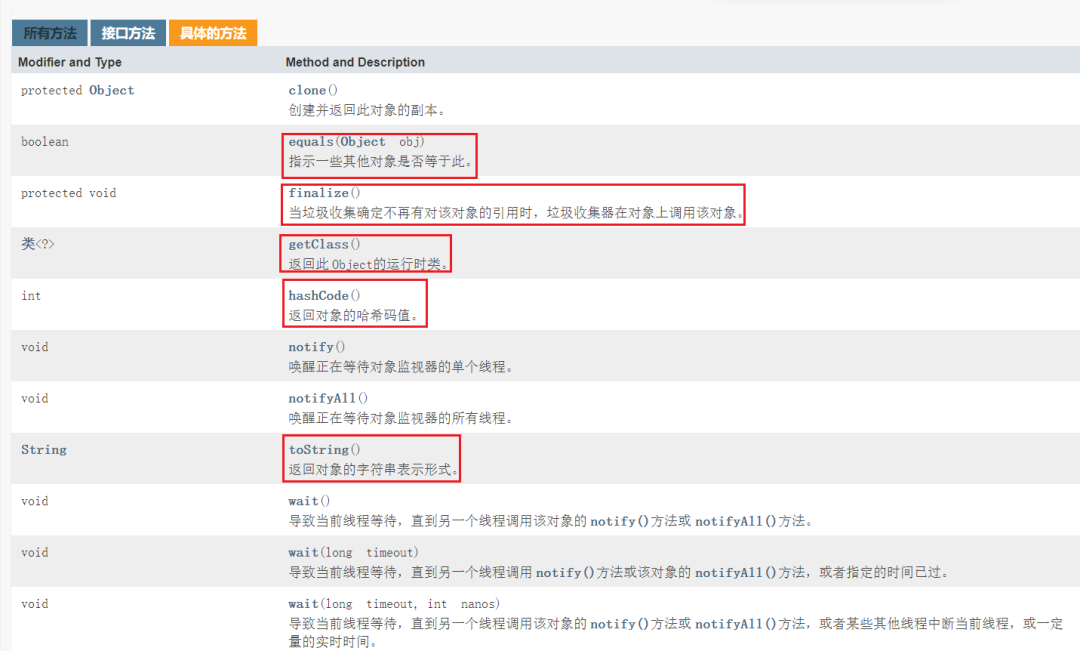#### ==和equals区别

==是一个比较运算符

1. ==：既可以判断基本类型，又可以判断引用类型

2. ==：如果判断的是基本类型，判断的是值是否相等。

``````//==： 如果判断的是基本类型，判断的是 值 是否相等
int x1 = 10;
int x2 = 10;
double x3 = 10.0;
System.out.println(x1 == x2);//true
System.out.println(x1 == x3);//true
``````
1. ==：如果判断的是引用类型判断的是地址是否相等，即判断是不是同一个对象
``````package Equals;

public class Test01 {

public static void main(String[] args) {

//==： 如果判断的是引用类型，判断的是地址是否相等，即判断是不是同一个对象
A a = new A();
A b = a;
A c = b;
System.out.println(a==c);// ? true
System.out.println(b==c);// true

B obj = a;
System.out.println(obj==c);// true

}
}

class B{

}
class A extends B{

}
``````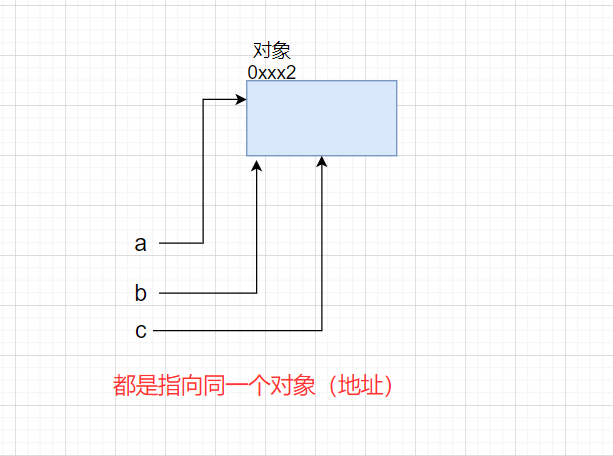1. equals方法是Object类中的方法，只能判断引用类型

2. equals默认判断的是地址是否相等，子类(Object类是所有类的父类)往往重写该方法，用于判断内容是否相等

``````//Object类 equals()方法原码

//默认判断地址是否一样
public boolean equals(Object obj) {

return (this == obj);
}

public boolean equals(Object anObject) {

if (this == anObject) {

// 如果是同一个对象(地址相同)
return true; // 返回true
}
if (anObject instanceof String) {

// 判断类型
String anotherString = (String)anObject; // 向下转型
int n = value.length;
if (n == anotherString.value.length) {

// 如果长度相同
char v1[] = value;
char v2[] = anotherString.value;
int i = 0;
while (n-- != 0) {

// 比较每一个字符
if (v1[i] != v2[i])
return false;
i++;
}
return true; // 如果两个字符串每一个字符都相同，则返回true
}
}
return false;
}

``````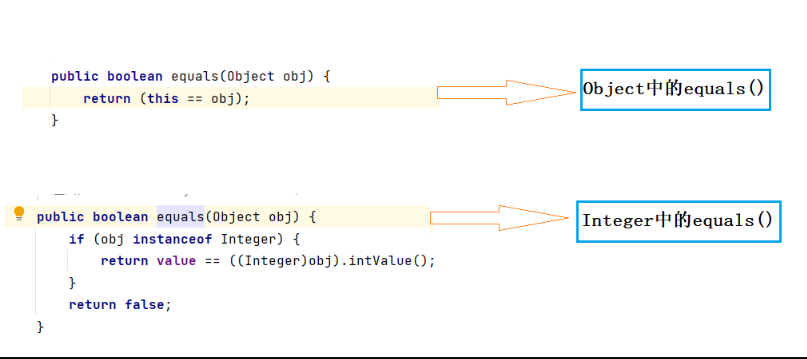【小练习】

``````package Equals;

public class EqualsTest01 {

public static void main(String[] args) {

Person p1 = new Person();
p1.name = "tom";
Person p2 = new Person();
p2.name = "tom";

System.out.println(p1 == p2);// 引用类型——判断是否为同一个对象（地址）
System.out.println(p1.name.equals(p2.name));// p.name是String类型，重写了equals()方法——判断内容是否一样
System.out.println(p1.equals(p2));//p1,p2属于Person类，该类并没有重写equals()方法（继承父类equals()方法，即判断地址）

String s1 = new String("abc");
String s2 = new String("abc");

System.out.println(s1.equals(s2));
System.out.println(s1 == s2);

}
}

class Person{

public String name;
}

``````

``````false
true
false
true
false
``````

#### hashCode方法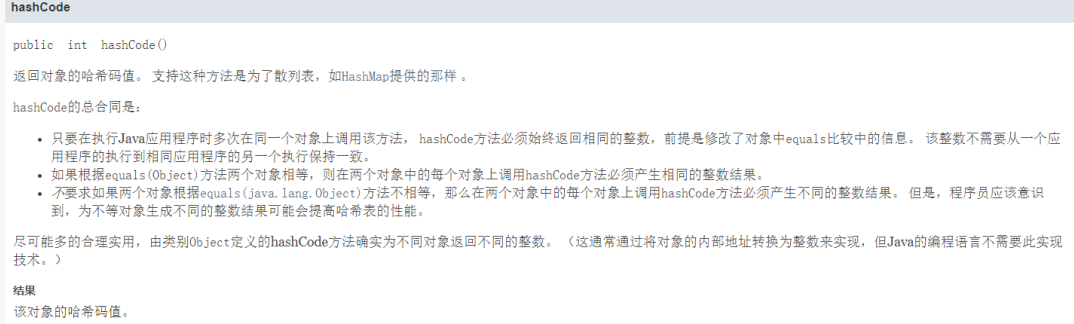1. 提高具有哈希结构的容器的效率
2. 两个引用，如果指向的是同一个对象，则哈希值肯定一样
3. 两个引用，如果指向的是不同对象，则哈希值是不一样的
4. 哈希值主要根据地址号来！不能将哈希值完全等价于地址
5. 在后面的集合中hashCode如果需要的话，也会重写
``````package hashCode;

public class HashCode {

public static void main(String[] args) {

AA aa = new AA();
AA aa2 = new AA();
AA aa3 = aa;
System.out.println("aa.hashCode()="+ aa.hashCode());
System.out.println("aa2.hashCode()="+ aa2.hashCode());
System.out.println("aa3.hashCode()="+ aa3.hashCode());
}
}

class AA{

}
``````

``````aa.hashCode()=460141958
aa2.hashCode()=1163157884
aa3.hashCode()=460141958
``````

#### toString方法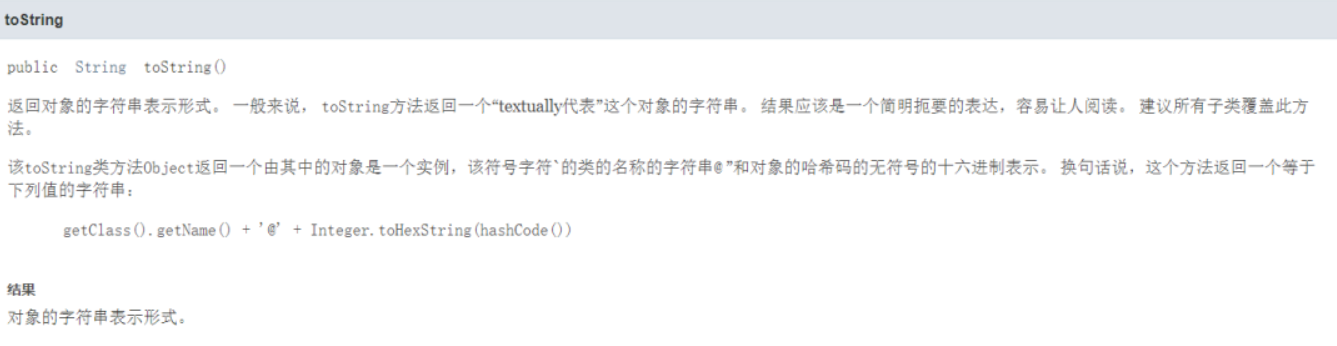``````/*
Object toString()原码
//(1)getClass().getName() 类的全类名（包名+类名）
//(2)Integer.toHexString(hashCode()) 将hashCode的值转成16进制字符串
public String toString() {
return getClass().getName() + "@" + Integer.toHexString(hashCode());
}
*/
``````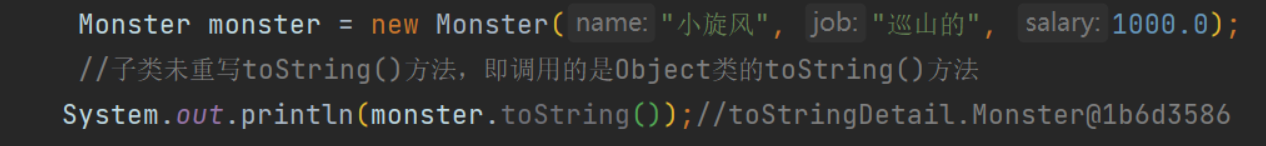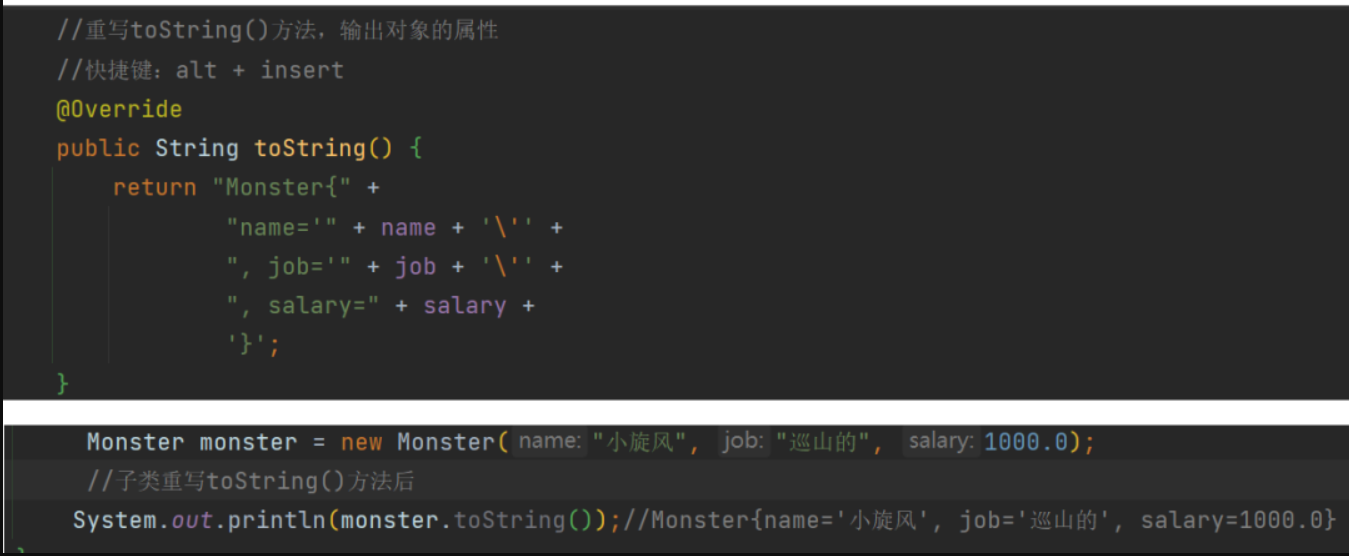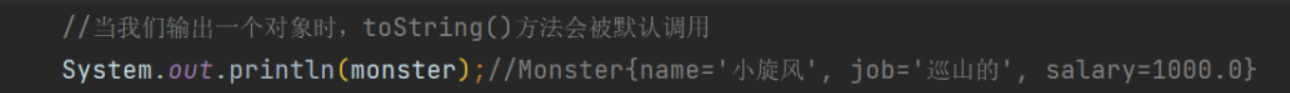#### finzlize方法（不常用）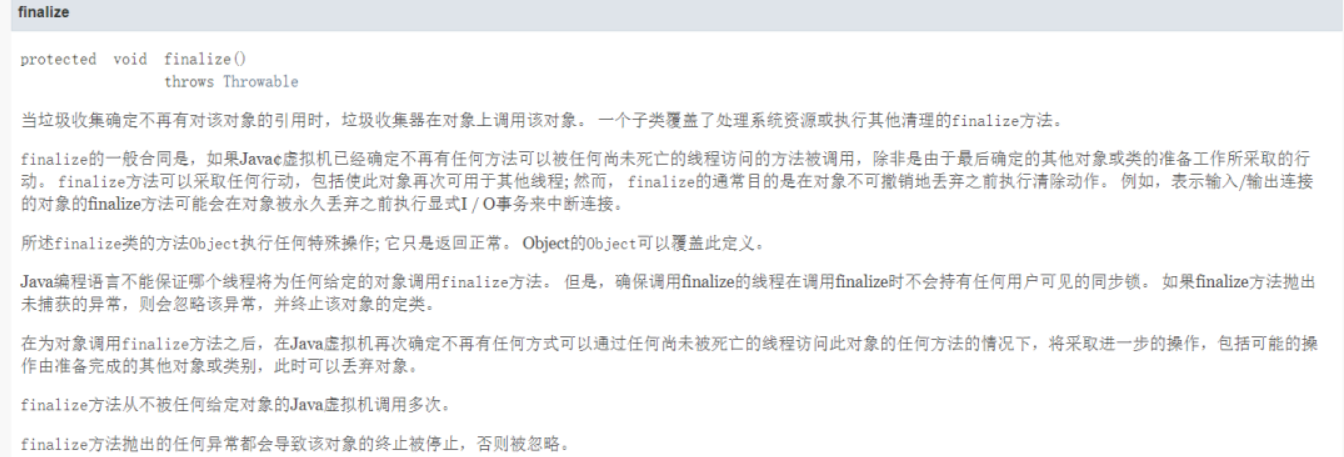finzlize方法：当垃圾收集确定不再有对该对象的引用时，垃圾收集器在对象上调用该对象。

1. 当对象被回收时，系统自动调用该对象的finzlize方法。子类可以重写该方法，做一些释放资源的操作
2. 什么时候被回收：当某个对象没有任何引用时，则jvm就认为这个对象是一个垃圾对象，就会时候垃圾回收机制来销毁该对象，在销毁该对象前，会先调用finzlize方法。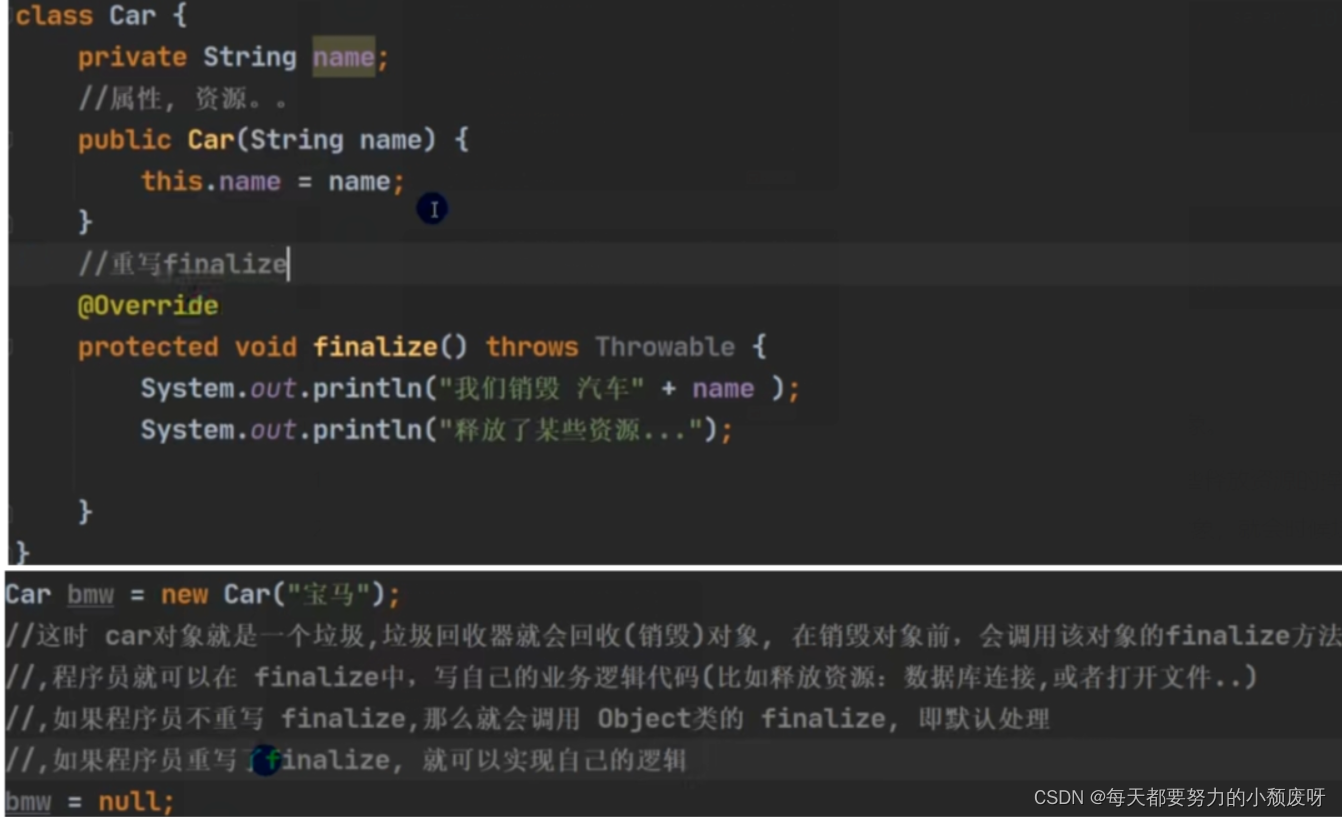>
垃圾回收机制的调用，是由系统来决定（即有自己的GC算法），也可以通过System.gc()主动触发垃圾回收机制。

### 10.4 包装类

1.基本数据类型以及对应的包装类对应关系如下：
byte -> Byte

short -> Short

int -> Integer

long -> Long

float -> Float

double -> Double

char -> Character

boolean -> Boolean

2.包装类的意义：

3.包装类常用方法：
Integer.parseInt()

Long.paseLong()

Double.parseDouble()

``````public class Test {

public static void main(String[] args) {

//        Integer i = new Integer(10);// 创建包装类对象
//        Integer ii = 10; // 自动打包
//        System.out.println(i+10); // 在使用上，int 和Integer 其实没有区别，可以互相使用
//        System.out.println(ii+10);
//        int j = ii;// 自动解包
//        System.out.println(j+100);

String a = "12";
String b = "34";
System.out.println(a+b); // 1234
// 转型:
// 字符串转成int的唯一方案
int c = Integer.parseInt(a);
int d = Integer.parseInt(b);
System.out.println(c+d); // 46

// 字符串转成double类型
String e = "1.25";
double f = Double.parseDouble(e);
System.out.println(f*6); // 7.5

// 转成long类型
long l = Long.parseLong("1234567");
System.out.println(l);
}
}

``````

### 10.5 DecimalFormat类

DecimalFormat：对小数进行格式化，保留几位小数。

. 表示小数点

0和# 表示数位，保留几位就几个0或者#

``````import java.text.DecimalFormat;
import java.util.Scanner;

public class Test {

public static void main(String[] args) {

double d= 10/3.0;
System.out.println(d);//3.3333333333333335

// . 表示小数点
// 0和#表示数字

// 保留两位小数                        格式
DecimalFormat df = new DecimalFormat(".00"); // 或者.##
String s = df.format(d); // 把 d 转成上面设置的格式
System.out.println(s);//3.33

}
}

``````

### 10.6 数学类

1. abs()：获取绝对值
2. max()：求最大值
3. min()：求最小值
4. pow()：求次幂
5. round()：四舍五入
6. sqrt()：求平方根

### 10.7 时间类

Java常用时间类：

1. Date 日期类

2. Calendar 日历类

3. SimpleDateFormat 格式化时间类

Date和Calendar类 在java.util包中

SimpleDateFormat类 在java.text包

#### Date 日期

【1】new Date() 可以获取到系统时间

【2】getTime() 能获取到时间的long形式，可以用来计算时间差

getTime()——获取计算机底层存储的数字，返回一个数字用来表示时间，这个数字的类型long，单位为毫秒。

``````import java.util.Date;

public class Test {

public static void main(String[] args) {

Date d = new Date();
System.out.println(d); // 系统时间
//get...()——获取年月日.....
System.out.println(d.getYear()+1900); // 从1900年开始算的
System.out.println(d.getMonth()+1); // 月份从0开始计算
System.out.println(d.getDate()); // 天数
System.out.println(d.getHours());// 小时

//getTime()——获取到时间的毫秒形式 返回的是long
System.out.println(d.getTime());
}
}
``````

#### Calendar 日历

【1】get() 获取到时间的某一部分

【2】set() 设置时间 - -> 计算时间：系统已经帮我们设置好了，不用担心二月有多少天等问题，计算时间十分方便

Calendar rightNow = Calendar.getInstance();

【参考代码】：get() 获取到时间的某一部分：

``````package date;

import java.util.Calendar;

public class TestCalendar {

public static void main(String[] args) {

Calendar cal = Calendar.getInstance();
//        System.out.println(cal);

/*
假设当天：
2022
5
10
*/
cal.set(Calendar.DATE,cal.get(Calendar.DATE)+31); // 计算时间(这里用天数计算的)

// 获取Calendar创建的对象里的所有内容
System.out.println(cal.get(Calendar.YEAR)); //  年
System.out.println(cal.get(Calendar.MONTH)+1); // 月份
System.out.println(cal.get(Calendar.DATE)); // 日
System.out.println(cal.get(Calendar.HOUR_OF_DAY));// 小时
System.out.println(cal.get(Calendar.MINUTE));
System.out.println(cal.get(Calendar.SECOND));
}
}

``````

【参考代码】：set() 设置时间 - -> 计算时间：

``````package date;

import java.util.Calendar;
import java.util.Date;

public class TestCalendar {

public static void main(String[] args) {

Date d = new Date();

Calendar cal = Calendar.getInstance();

cal.setTime(d);// 把Date转化成Calendar

System.out.println(cal);
System.out.println(cal.get(Calendar.YEAR)); //  年
System.out.println(cal.get(Calendar.MONTH)+1); // 月份：从0开始的
System.out.println(cal.get(Calendar.DATE)); // 日

}
}

``````

#### SimpleDateFormat格式化时间

Date，Calendar通过引用也可以进行时间的格式化，但比较繁琐，而SimpleDateFormat类是专门帮我们格式化时间的工具类，它在java.text包中。

【时间格式】：yyyy-MM-dd HH:mm:ss

SimpleDateFormat类有两大常用方法：

【1】format(Date)：

format(Date) 帮我们把时间转成字符串，字符串的格式为SimpleDateFormat类定义对象时设置的时间格式

``````package Simple;

import java.text.SimpleDateFormat;
import java.util.Date;
import java.util.logging.SimpleFormatter;

public class Test {

public static void main(String[] args) {

Date d = new Date();
System.out.println(d); //Thu Aug 12 08:40:08 CST 2021  不美观

//                  设置格式化时间的模式，我们常用yyyy-MM-dd HH:mm:ss这个模式
SimpleDateFormat sdf = new SimpleDateFormat("yyyy-MM-dd HH:mm:ss");// 时间格式
String s = sdf.format(d); // 格式化时间
System.out.println(s); // 2021-08-12 08:45:09
}
}
``````

【2】parse(String)：

parse(String) 帮我们把字符串转化成时间

``````package Simple;

import java.text.ParseException;
import java.text.SimpleDateFormat;
import java.util.Date;
import java.util.Scanner;

public class Test2 {
public static void main(String[] args) throws ParseException {
Scanner sc = new Scanner(System.in);

System.out.println("请输入一个时间(yyyy-MM-dd HH:mm:ss)：");

String s = sc.nextLine();

SimpleDateFormat sdf = new SimpleDateFormat("yyyy-MM-dd HH:mm:ss");

Date d =  sdf.parse(s); // 把字符串转成时间

System.out.println(d);

}
}
``````

#### 计算时间差

1. 格式化时间
2. 先将字符串转化成long类型时间
3. 计算毫秒级别时间差，取绝对值
4. 毫秒级别时间差转成秒级别
5. 秒级别时间差转成分钟级别
6. 分钟级别时间差转化显示成xx小时xx分钟

``````package Simple;

import java.text.ParseException;
import java.text.SimpleDateFormat;
import java.util.Date;

public class TestDiff {

public static void main(String[] args) throws ParseException {

String s1 = "2021-05-20 12:00:00"; // 开始时间
String s2 = "2022-5-10 14:35:00"; // 结束时间

//格式化时间
SimpleDateFormat sdf = new SimpleDateFormat("YYYY-MM-dd HH:mm:ss");

//将字符串转成时间形式
Date d1 = sdf.parse(s1);
Date d2 = sdf.parse(s2);

//计算时间差:先要获取时间毫秒形式（long类型） 再做差
long long1 = d1.getTime();
long long2 = d2.getTime();
long diffTime = Math.abs(long1 - long2);

// 秒级别时间差
long diffSec = diffTime / 1000;

// 分级别时间差
long diffMin = diffSec / 60;

//显示 xx小时xx分钟
long displayHours = diffMin / 60; // 小时
long displayMin = diffMin % 60; //分钟

System.out.println("您学习的时长为："+displayHours+"小时"+displayMin+"分钟");

}
}
``````

### 10.8 内部类

1.成员内部类（非静态内部类）

``````package NeiBuLei;

public class OuterClass {

//成员变量
private String OuterName;
//成员方法
public void display(){

System.out.println("这是外部类方法！");
System.out.println(OuterName);
}
//内部类
public class InnerClass{

//成员变量
private String InnerNme;
//构造方法
public InnerClass() {

InnerNme = "Inner Class";
}
//成员方法
public void display(){

System.out.println("这是内部类方法！");
System.out.println(InnerNme);
}
}
// 主方法
public static void main(String[] args) {

OuterClass outerClass = new OuterClass();
outerClass.display();//这是外部类方法！null

// 这个类是内部类，已经不是独立的类了，因此不能像外部类一样直接创建！
//InnerClass innerClass = new InnerClass(); 行不通
OuterClass.InnerClass innerClass = outerClass.new InnerClass();// 同成员方法/变量 只是加了个前缀
innerClass.display();// 这是内部类方法！
}

}

``````

``````这是外部类方法！
null

Inner Class
``````

2.局部内部类

``````package NeiBuLei;

public class OuterClass {

//成员变量
private String OuterName;
//成员方法
public void display(){

class InnerClass {

public void print(){

System.out.println("这是一个局部内部类方法！");
}
}
InnerClass innerClass = new InnerClass();
innerClass.print();
}

// 主方法
public static void main(String[] args) {

OuterClass outerClass = new OuterClass();
outerClass.display();
}
}
``````

3.静态内部类

``````package NeiBuLei;

public class OuterClass {

//成员变量
private String OuterName;
//成员方法
public void display(){

System.out.println("这是外部类方法！");
System.out.println(OuterName);
}
//静态内部类
public static class InnerClass{

private String InnerName;

public InnerClass() {

InnerName = "Inner Class";
}

//成员方法
public void display(){

System.out.println("这是静态内部类方法！");
System.out.println(InnerName);
}
}

// 主方法
public static void main(String[] args) {

OuterClass outerClass = new OuterClass();
outerClass.display();
// 静态内部类的构造不依赖与外部类，可以直接通过类本身进行构造！
InnerClass innerClass = new InnerClass();
innerClass.display();
}

}
``````

``````这是外部类方法！
null

Inner Class
``````

4.匿名内部类

``````package NeiBuLei;

public interface MyInterface {

public void test();
}
``````

``````package NeiBuLei;

public class MyImplement implements MyInterface{

@Override
public void test() {

System.out.println("test");
}
}
``````

``````package NeiBuLei;

public class Test {

public static void main(String[] args) {

//实现类
MyInterface myInterface = new MyImplement();
myInterface.test();

//匿名内部类
MyInterface myInterface1 = new MyInterface() {

// 接口是不能new的，这里new的是接口的实现类（和实现类是一样的（同一个东西）,没有实例而已）
@Override
public void test() {

System.out.println("test");
}
};
myInterface.test();
/**
* 最终两种实现方式的结果都是一样的！
*/
}
}
``````